Model Fit Statistics

Suppose the model contains p regression parameters. Let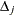and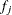be the event indicator and the frequency, respectively, of the jth observation. The three criteria displayed by the PHREG procedure are calculated as follows:

• –2 Log Likelihood: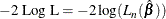where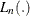is a partial likelihood function for the corresponding TIES= option as described in the section Partial Likelihood Function for the Cox Model, and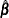is the maximum likelihood estimate of the regression parameter vector.

• Akaike’s Information Criterion: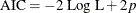• Schwarz Bayesian (Information) Criterion: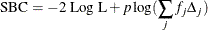The –2 Log Likelihood statistic has a chi-square distribution under the null hypothesis (that all the explanatory effects in the model are zero) and the procedure produces a p-value for this statistic. The AIC and SBC statistics give two different ways of adjusting the –2 Log Likelihood statistic for the number of terms in the model and the number of observations used. These statistics should be used when comparing different models for the same data (for example, when you use the METHOD=STEPWISE option in the MODEL statement); lower values of the statistic indicate a more desirable model.